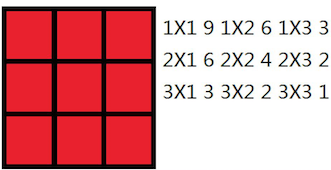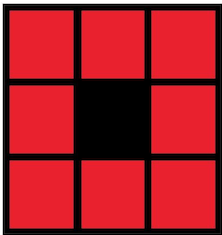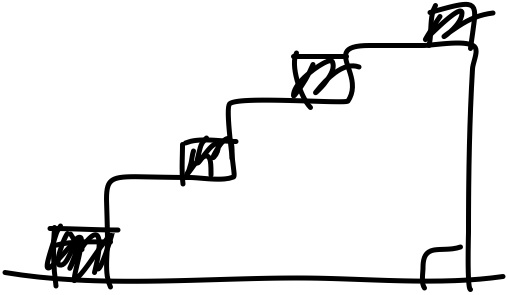# ACM-ICPC 2018 南京赛区网络预赛

An Olympian Math Problem
•  54.76%
•  1000ms
•  65536K

Alice, a student of grade 6

We denote k!

k! = 1 \times 2 \times \cdots \times (k - 1) \times k

We denote S

S = 1 \times 1! + 2 \times 2! + \cdots +

Then S

You are given an integer n

You have to calculate S

### Input

The first line contains an integer T(T \le 1000)

For each test case, there is a line which has an integer n

It is guaranteed that 2 \le n\le 10^{18}

### Output

For each test case, print an integer S

### Hint

The first test is: S = 1\times 1!= 1

The second test is: S = 1\times 1!+2 \times 2!= 5

#### 样例输入

2
2
3

#### 样例输出

1
2

#### 题目来源

ACM-ICPC 2018 南京赛区网络预赛

A题在抢首A的道路上努力奔跑，然后CE

#include<stdio.h>
#include<bits/stdc++.h>
using namespace std;
#define lson l,(l+r)/2,rt<<1
#define rson (l+r)/2+1,r,rt<<1|1
#define dbg(x) cout<<#x<<" = "<< (x)<< endl
#define pb push_back
#define fi first
#define se second
#define ll long long
#define sz(x) (int)(x).size()
#define pll pair<long long,long long>
#define pii pair<int,int>
#define pq priority_queue
const int N=1e5+5,MD=1e9+7,INF=0x3f3f3f3f;
const ll LL_INF=0x3f3f3f3f3f3f3f3f;
const double eps=1e-9,e=exp(1),PI=acos(-1.);
int main()
{
ios::sync_with_stdio(false),cin.tie(0),cout.tie(0);
int T;
cin>>T;
while(T--)
{
ll n;
cin>>n;
cout<<n-1<<"\n";
}
return 0;
}

The writing on the wall

Feeling hungry, a cute hamster decides to order some take-away food (like fried chicken for only 30

However, his owner CXY thinks that take-away food is unhealthy and expensive. So she demands her hamster to fulfill a mission before ordering the take-away food. Then she brings the hamster to a wall.

The wall is covered by square ceramic tiles, which can be regarded as a n * m

For example, the following 3 * 3Such problem is quite easy for little hamster to solve, and he quickly manages to get the answer.

Seeing this, the evil girl CXY picks up a brush and paint some tiles into black, claiming that only those rectangles which don't contain any black tiles are valid and the poor hamster should only calculate the number of the valid rectangles. Now the hamster feels the problem is too difficult for him to solve, so he decides to turn to your help. Please help this little hamster solve the problem so that he can enjoy his favorite fried chicken.

### Input

There are multiple test cases in the input data.

The first line contains a integer T

For each test case, the first line contains 3

For the next k

For all the test cases,

1 \le n \le 10^5,1\le m \le 100

0 \le k \le 10^5 , 1 \le x \le n, 1 \le y \le m

It's guaranteed that at most 2

### Output

For each test case, print "Case #x

### Hint

The second test case looks as follows:#### 样例输入

2
3 3 0
3 3 1
2 2

#### 样例输出

Case #1: 36
Case #2: 20

#### 题目来源

ACM-ICPC 2018 南京赛区网络预赛

B是个51nod的原题，可以用单调栈维护，维护出这样一个玩意#include<stdio.h>
#include<bits/stdc++.h>
using namespace std;
#define lson l,(l+r)/2,rt<<1
#define rson (l+r)/2+1,r,rt<<1|1
#define dbg(x) cout<<#x<<" = "<< (x)<< endl
#define pb push_back
#define fi first
#define se second
#define ll long long
#define sz(x) (int)(x).size()
#define pll pair<long long,long long>
#define pii pair<int,int>
#define pq priority_queue
const int N=1e5+5,MD=1e9+7,INF=0x3f3f3f3f;
const ll LL_INF=0x3f3f3f3f3f3f3f3f;
const double eps=1e-9,e=exp(1),PI=acos(-1.);
using namespace std;
bool s[N];
ll a[N];
int b[N],sta[N],L[N],R[N],T[N];
int n, m;
int main()
{
ios::sync_with_stdio(false),cin.tie(0),cout.tie(0);
int cc;
cin>>cc;
for(int ca=1;ca<=cc;ca++)
{
int n,m,k;
cin>>n>>m>>k;
for(int i=1; i<=n; i++)for(int j=1; j<=m; j++)s[i][j]=1;
for(int i=0,x,y; i<k; i++)cin>>x>>y,s[x][y]=0;
memset(a,0,sizeof a),memset(b,0,sizeof b);
b=b[m+1]=-1;
for(int i=1,tot; i<=n; i++)
{
tot=-1;
sta[++tot]=0;
for(int j=1; j<=m; j++)
{
if(s[i][j]==1)++b[j];
else b[j]=0;
while(b[sta[tot]]>b[j])--tot;
T[j]=sta[tot];
while(b[sta[tot]]>=b[j])--tot;
L[j]=sta[tot],sta[++tot]=j;
}
tot=-1;
sta[++tot]=m+1;
for(int j=m; j>0; j--)
{
while(b[sta[tot]]>=b[j])--tot;
R[j]=sta[tot],sta[++tot]=j;
}
for(int j=1,s,t; j<=m; j++)
{
if(b[j]&&b[T[j]]!=b[j])
{
s=max(1,max(b[L[j]],b[R[j]])+1),t=R[j]-L[j]-1;
if(s>b[j]) continue;
++a[s][t],--a[b[j]+1][t];
}
}
}
ll ans=0,tt,t;
for(int i=1; i<=n; i++)for(int j=1; j<=m; j++)a[i][j]+=a[i-1][j];
for(int i=1; i<=n; i++)
{
tt=0;
for(int j=m; j>0; j--)t=a[i][j],a[i][j]+=a[i][j+1]+tt,tt+=t;
for(int j=1; j<=m; j++)ans+=a[i][j];
}
cout<<"Case #"<<ca<<": "<<ans<<"\n";
}
return 0;
}

AC Challenge

Dlsj is competing in a contest with n (0 < n \le 20)

However, he can submit i

"I wonder if I can leave the contest arena when the problems are too easy for me."
"No problem."
—— CCF NOI Problem set

If he submits and passes the i

Your task is to calculate the maximum number of points he can get in the contest.

### Input

The first line of input contains an integer, n

Then follows n

### Output

Output one line with one integer, the maximum number of points he can get in the contest.

### Hint

In the first sample.

On the first minute, Dlsj submitted the first problem, and get 1 \times 5 + 6 = 11

On the second minute, Dlsj submitted the second problem, and get 2 \times 4 + 5 = 13

On the third minute, Dlsj submitted the third problem, and get 3 \times 3 + 4 = 13

On the forth minute, Dlsj submitted the forth problem, and get 4 \times 2 + 3 = 11

On the fifth minute, Dlsj submitted the fifth problem, and get 5 \times 1 + 2 = 7

So he can get 11+13+13+11+7=55

In the second sample, you should note that he doesn't have to solve all the problems.

#### 样例输入1

5
5 6 0
4 5 1 1
3 4 1 2
2 3 1 3
1 2 1 4

#### 样例输出1

55

#### 样例输入2

1
-100 0 0

#### 样例输出2

0

#### 题目来源

ACM-ICPC 2018 南京赛区网络预赛

#include <bits/stdc++.h>
using namespace std;
int dp[1<<20],a,b,S;
int f(int x)
{
int ans=0;
while(x)
{
x-=x&-x;
ans++;
}
return ans;
}
int main()
{
int n;
scanf("%d",&n);
for(int i=0,m;i<n;i++)
{
scanf("%d%d%d",&a[i],&b[i],&m);
for(int j=0,x;j<m;j++)
scanf("%d",&x),S[i]|=(1<<(x-1));
}
//for(int i=0;i<n;i++)
//    printf("%d\n",S[i]);
memset(dp,-1,sizeof dp);
dp=0;
for(int s=0;s<1<<n;s++)
{
if(dp[s]==-1) continue;
for(int i=0;i<n;i++)
if((s&S[i])==S[i]&&(s&(1<<i))==0)
dp[s|(1<<i)]=max(dp[s|(1<<i)],dp[s]+a[i]*(f(s)+1)+b[i]);
}
int ans=0;
for(int i=0;i<1<<n;i++)
ans=max(ans,dp[i]);
printf("%d\n",ans);
return 0;
}
Sum
•  26.14%
•  1000ms
• 512000K

A square-free integer is an integer which is indivisible by any square number except 1

### Input

The first line contains an integer T(T\le 20)

For each test case, there first line has a integer n(n \le 2\cdot 10^7)

### Output

For each test case, print the answer \sum_{i = 1}^n f(i)

### Hint

\sum_{i = 1}^8 f(i)=f(1)+ \cdots +f(8)

#### 样例输入

2
5
8

#### 样例输出

8
14

#### 题目来源

ACM-ICPC 2018 南京赛区网络预赛

#include<bits/stdc++.h>
using namespace std;
typedef long long ll;
const int N=2e7+5;
bool pri[N];
int prime[N];
ll f[N];
int tot=0;
int main()
{
pri=1,f=1;
for(int i=2; i<N; i++)
{
if(pri[i]==0)prime[tot++]=i,f[i]=2;
for(int j=0,num; j<tot&&i*1LL*prime[j]<N; ++j)
{
num=i*prime[j],pri[num]=1;
if(i%prime[j])f[num]=f[i]*2;
else if(i%(1LL*prime[j]*prime[j])==0)f[num]=0;
else
{
f[num]=f[num/prime[j]/prime[j]];
break;
}
}
}
for(int i=2; i<N; i++)f[i]+=f[i-1];
int t,n;
scanf("%d",&t);
while(t--)scanf("%d",&n),printf("%lld\n",f[n]);
}

#include<stdio.h>
#include<bits/stdc++.h>
using namespace std;
#define lson l,(l+r)/2,rt<<1
#define rson (l+r)/2+1,r,rt<<1|1
#define dbg(x) cout<<#x<<" = "<< (x)<< endl
#define pb push_back
#define fi first
#define se second
#define ll long long
#define sz(x) (int)(x).size()
#define pll pair<long long,long long>
#define pii pair<int,int>
#define pq priority_queue
const int N=2e7+5,MD=1e9+7,INF=0x3f3f3f3f;
const ll LL_INF=0x3f3f3f3f3f3f3f3f;
const double eps=1e-9,e=exp(1),PI=acos(-1.);
int a[N];
int main()
{
ios::sync_with_stdio(false),cin.tie(0),cout.tie(0);
for(int i=2; i*i<N; i++)
for(int j=i*i; j<N; j+=i*i)a[j]=1;
for(int i=1; i<N; i++)a[i]+=a[i-1];
int T;
cin>>T;
while(T--)
{
int n;
cin>>n;
ll ans=0;
int l=1,r;
while(l<=n)
{
r=n/(n/l)+1;
ans+=1LL*(r-l)*(n/l)-2LL*(a[r-1]-a[l-1])*(n/l)+1LL*(a[r-1]-a[l-1])*a[n/l];
l=r;
}
cout<<ans<<"\n";
}
return 0;
}

Magical Girl Haze

There are N

### Input

The first line has one integer T(1 \le T\le 5)

For each test case, the first line has three integers N, M

Then the following M

It is guaranteed that N \le 100000, M \le 200000, K \le 10

### Output

For each test case, print the minimum distance.

#### 样例输入

1
5 6 1
1 2 2
1 3 4
2 4 3
3 4 1
3 5 6
4 5 2

#### 样例输出

3

#### 题目来源

ACM-ICPC 2018 南京赛区网络预赛

#include<bits/stdc++.h>
using namespace std;

#define ll long long
const int maxn=1e5+5,maxm=2e5+5;
ll d[maxn];
int n,m,k;
struct edge
{
int v,next;
ll w;

}edges[maxm];
struct node
{
ll w;
int v;
bool operator<(const node &D)const{
return w>D.w;
}
};
{
char in;bool IsN=false;
in=getchar();
if(in==EOF) return false;
while(in!='-'&&(in<'0'||in>'9')) in=getchar();
if(in=='-')
{
IsN=true;
num=0;
}
else num=in-'0';
while(in=getchar(),in>='0'&&in<='9')
{
num*=10,num+=in-'0';
}
if(IsN) num=-num;
return true;
}
void dij()
{
memset(d,0x3f,sizeof d);
priority_queue<node>q;
q.push({0,1});
d=0;
while(!q.empty())
{
auto u=q.top();q.pop();
if(u.v==n)return;
{
edge &v=edges[i];
for(int j=0;j<=k;j++)
{
if(j>=1&&d[j][v.v]>d[j-1][u.v])
q.push({d[j][v.v]=d[j-1][u.v],v.v});
if(d[j][v.v]>d[j][u.v]+v.w)
q.push({d[j][v.v]=d[j][u.v]+v.w,v.v});
}
}
}
}
int main()
{
int t;
while(t--)
{
cnt=0;
for(int i=0,u,v,w;i<m;i++)
{
edges[cnt].v=v;
edges[cnt].w=1LL*w;
}